Question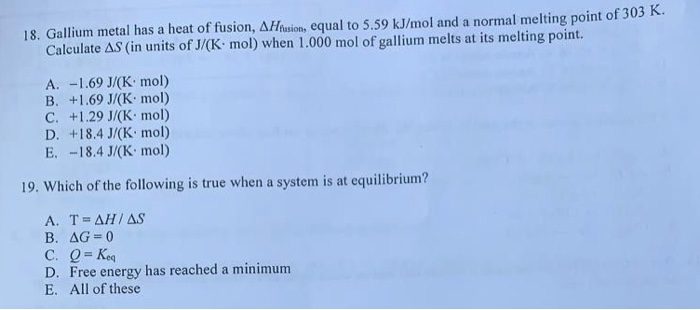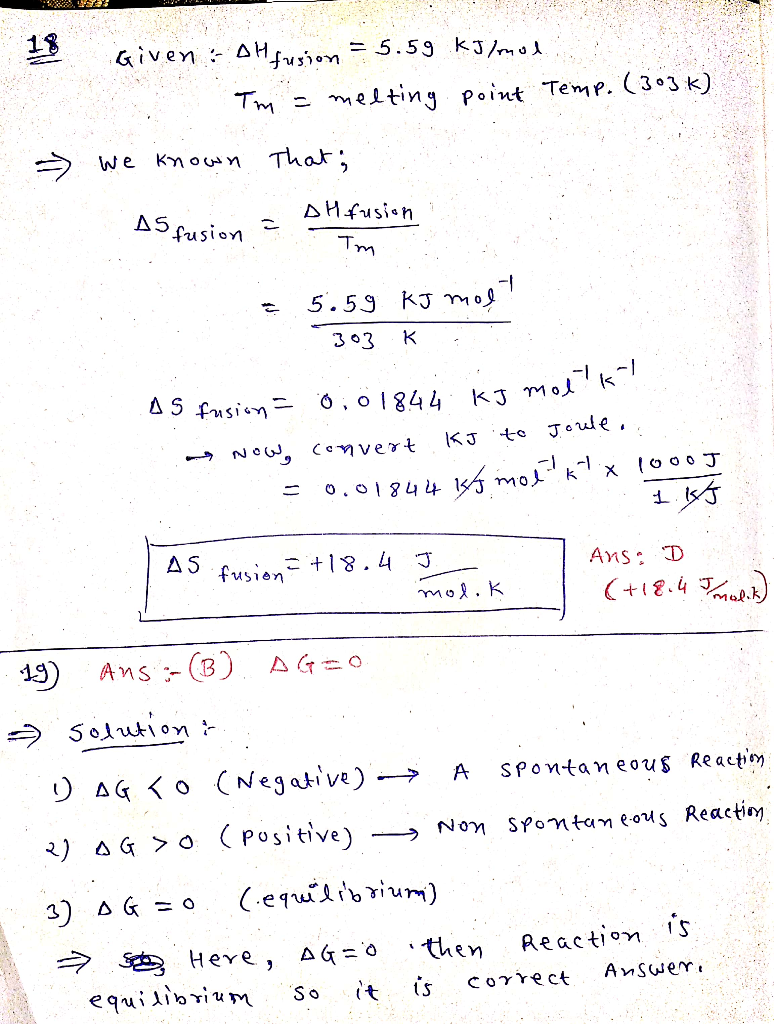#### Earn Coins

Coins can be redeemed for fabulous gifts.

Similar Homework Help Questions
• ### Gallium has a melting point of 29.8oC and an enthalpy of fusion of 5.59 kJ/mol. Calculate...

Gallium has a melting point of 29.8oC and an enthalpy of fusion of 5.59 kJ/mol. Calculate the change in entropy of the system, surroundings, and universe to show that the following process is spontaneous if 10.0 grams of liquid gallium is left in a 20.0oC room.Ga (l, 29.8oC) --> Ga (s, 29.8oC)

• ### 14. For bismuth, Bi, the heat of fusion at its normal melting point of 271 °C...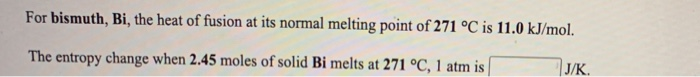14. For bismuth, Bi, the heat of fusion at its normal melting point of 271 °C is 11.0 kJ/mol. The entropy change when 2.45 moles of solid Bi melts at 271 °C, 1 atm is J/K. For magnesium, Mg, the heat of fusion at its normal melting point of 649 °C is 9.0 kJ/mol. The entropy change when 1.72 moles of liquid Mg freezes at 649 °C, 1 atm is J/K

• ### For ethanol, C2H5OH, the heat of fusion at its normal melting point of -115 °C is...

For ethanol, C2H5OH, the heat of fusion at its normal melting point of -115 °C is 5.0 kJ/mol. The entropy change when 1.84 moles of solid C2H5OH melts at -115 °C, 1 atm is J/K.

• ### For copper, Cu, the heat of fusion at its normal melting point of 1083 °C is...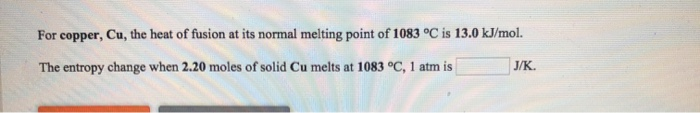For copper, Cu, the heat of fusion at its normal melting point of 1083 °C is 13.0 kJ/mol. The entropy change when 2.20 moles of solid Cu melts at 1083 °C, 1 atm is J/K

• ### The enthalpy of fusion for benzene (C6H6) is 9.937 kJ/mol, and the entropy change for fusion...

The enthalpy of fusion for benzene (C6H6) is 9.937 kJ/mol, and the entropy change for fusion is 35.7 J/(mol K). What is the normal melting point for benzene in degree Celsius?

• ### What is the heat of fusion of chromium (in kJ/mol) if 20.0 grams of solid chromium absorb 5.82 ?...

What is the heat of fusion of chromium (in kJ/mol) if 20.0 grams of solid chromium absorb 5.82 ? 103 J of heat in melting at 2173 K, its melting point?

• ### The enthalpy of fusion for benzene (C6H 6, 78.0 g/mol) is 127.40 kJ/kg, and its...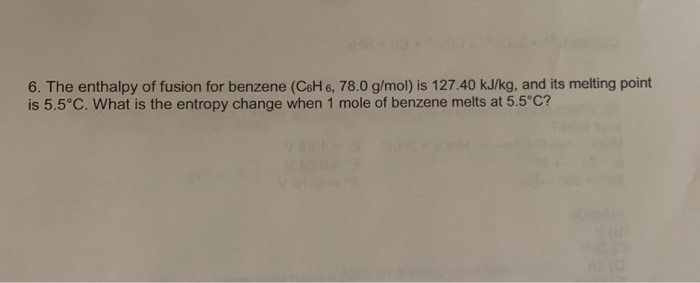The enthalpy of fusion for benzene (C6H6, 78.0 g/mol) is 127.40 kJ/kg, and its melting point is 5.5°C. What is the entropy change when 1 mole of benzene melts at 5.5°C?

• ### 6. The melting point of CaTiSiOs is 1400°C and the heat of fusion at the normal melting point is 123 700 J mol-1. Calculate the heat of fusion at 1300°C. Cnsolid = 177.4 + 23.2 x 10', 40.3 x...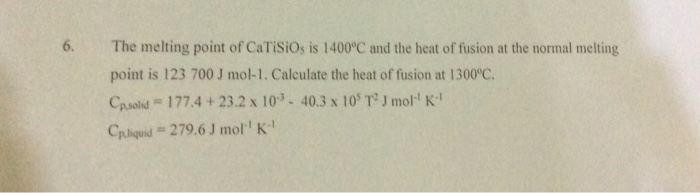6. The melting point of CaTiSiOs is 1400°C and the heat of fusion at the normal melting point is 123 700 J mol-1. Calculate the heat of fusion at 1300°C. Cnsolid = 177.4 + 23.2 x 10', 40.3 x 105 T2 J mor' K" Cphquid # 279.6 J mol'' K" 6. The melting point of CaTiSiOs is 1400°C and the heat of fusion at the normal melting point is 123 700 J mol-1. Calculate the heat of fusion at 1300°C....

• ### For benzene, C6H6, the heat of fusion at its normal melting point of 6 °C is...

For benzene, C6H6, the heat of fusion at its normal melting point of 6 °C is 10.0 kJ/mol. The entropy change when 1.86 moles of liquid C6H6 freezes at 6 °C, 1 atm is ? J/K.

• ### LUCIO C OVERS The heat of fusion AH, of benzene (CH) is 10.6 kJ/mol. Calculate the...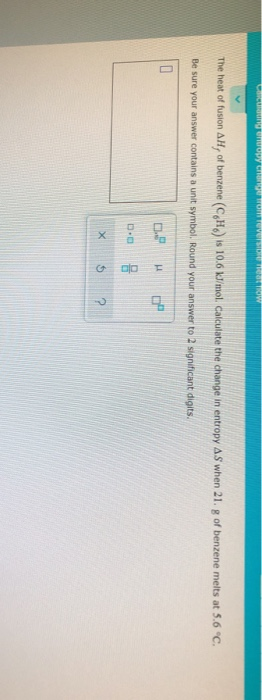LUCIO C OVERS The heat of fusion AH, of benzene (CH) is 10.6 kJ/mol. Calculate the change in entropy AS when 21. g of benzene melts at 5.6 °C. Be sure your answer contains a unit symbol. Round your answer to 2 significant digits. 0 0 Do X 5 ?